# Excel VBA : Filtering and copy pasting to new sheet or workbook

Suppose you are asked to apply filter on a column and paste result of a filter into a new worksheet or workbook and same process goes until all the unique values of the column are covered. In other words, this needs to be done for each unique values in a column in which we have applied filter. It is a very time consuming process if you do it manually. For example, you have a column in which there are 50 unique values. You have to do it 50 times which is a tedious and error-prone task. It can be easily done with Excel VBA programming.

The sample data is shown below :Filtering and Copying Data

How to Use
1. Open an Excel Workbook
2. Press Alt+F11 to open VBA Editor
3. Go to Insert Menu >> Module
4. In the module, paste the below program
5. Save the file as Macro Enabled Workbook (xlsm) or Excel 97-2003 Workbook (xls)
In the following excel macro, it is assumed a filter is applied on column F (Rank) and data starts from cell A1.

Excel Macro : Filter and Paste Unique Values to New Sheets

This macro would filter a column and paste distinct values to the sheets with their respective names. In this case, it creates four worksheets - 1 , 2, 3, 4 as these are unique values in column Rank (column F).
Sub filter()
Application.ScreenUpdating = False
Dim x As Range
Dim rng As Range
Dim last As Long
Dim sht As String

'specify sheet name in which the data is stored
sht = "DATA Sheet"

'change filter column in the following code
last = Sheets(sht).Cells(Rows.Count, "F").End(xlUp).Row
Set rng = Sheets(sht).Range("A1:F" & last)

Sheets(sht).Range("F1:F" & last).AdvancedFilter Action:=xlFilterCopy, CopyToRange:=Range("AA1"), Unique:=True

For Each x In Range([AA2], Cells(Rows.Count, "AA").End(xlUp))
With rng
.AutoFilter
.AutoFilter Field:=6, Criteria1:=x.Value
.SpecialCells(xlCellTypeVisible).Copy

ActiveSheet.Paste
End With
Next x

' Turn off filter
Sheets(sht).AutoFilterMode = False

With Application
.CutCopyMode = False
.ScreenUpdating = True
End With

End Sub
How to Filter and Paste Values to New Workbook

How to Customize the above program

1. Specify name of the sheet in which data is stored. Change the below line of code in the program.
sht = "DATA Sheet"
2. Change filter column (column F) and starting cell of range (A1) in the code.
last = Sheets(sht).Cells(Rows.Count, "F").End(xlUp).Row
Set rng = Sheets(sht).Range("A1:F" & last)
3. Starting cell of filter column - F1. Unique values of  column F are stored in column AA.
Sheets(sht).Range("F1:F" & last).AdvancedFilter Action:=xlFilterCopy, CopyToRange:=Range("AA1"), Unique:=True
For Each x In Range([AA2], Cells(Rows.Count, "AA").End(xlUp))
4. Change the value in this part of the code. In this case, 6 refers to column index number (i.e. Column F is 6th column).
.AutoFilter Field:=6, Criteria1:=x.Value
5. If you run the macro more than once, the error would occur as sheets already exist. To workaround this issue, follow the steps below -

(i) Add the following function before sub filter().
Function GetWorksheet(shtName As String) As Worksheet
On Error Resume Next
Set GetWorksheet = Worksheets(shtName)
End Function
(ii) Add the following lines of code after 'For Each x In Range([AA2], Cells(Rows.Count, "AA").End(xlUp))'. Caution : It removes the existing worksheets which were created via macro.
If Not GetWorksheet(x.Text) Is Nothing Then
Sheets(x.Text).Delete
End If
6. Suppose you want to copy specific columns instead of all the columns in your sheet. In the program below, it copies columns A, D and F
To change columns which need to be copied, make changes in this line of code -
Set rng1 = Union(.Range("A1:A" & last), .Range("D1:D" & last), .Range("F1:F" & last))

```Option Explicit

Function GetWorksheet(shtName As String) As Worksheet
On Error Resume Next
Set GetWorksheet = Worksheets(shtName)
End Function

Sub filter()
Application.ScreenUpdating = False
Dim x As Range
Dim rng As Range
Dim rng1 As Range
Dim last As Long
Dim sht As String

'Specify sheet name in which the data is stored
sht = "DATA Sheet"

'change filter column in the following code
last = Sheets(sht).Cells(Rows.Count, "F").End(xlUp).Row

With Sheets(sht)
Set rng = .Range("A1:F" & last)

'Specific columns which will be copied
Set rng1 = Union(.Range("A1:A" & last), .Range("D1:D" & last), .Range("F1:F" & last))

End With

Sheets(sht).Range("F1:F" & last).AdvancedFilter Action:=xlFilterCopy, CopyToRange:=Range("AA1"), Unique:=True

For Each x In Range([AA2], Cells(Rows.Count, "AA").End(xlUp))

If Not GetWorksheet(x.Text) Is Nothing Then
Sheets(x.Text).Delete
End If

With rng
.AutoFilter
.AutoFilter Field:=6, Criteria1:=x.Value
End With

With rng1
.SpecialCells(xlCellTypeVisible).Copy

ActiveSheet.Paste
End With
Next x

' Turn off filter
Sheets(sht).AutoFilterMode = False

With Application
.CutCopyMode = False
.ScreenUpdating = True
End With

End Sub
```
7. Suppose you want to filter and paste data to a new workbook instead of adding sheets in the same workbook.

```Option Explicit

Sub filter()
Application.ScreenUpdating = False
Dim x As Range
Dim rng As Range
Dim rng1 As Range
Dim last As Long
Dim sht As String
Dim newBook As Excel.Workbook
Dim Workbk As Excel.Workbook

'Specify sheet name in which the data is stored
sht = "DATA Sheet"

'Workbook where VBA code resides
Set Workbk = ThisWorkbook

'New Workbook
Workbk.Activate

'change filter column in the following code
last = Workbk.Sheets(sht).Cells(Rows.Count, "F").End(xlUp).Row

With Workbk.Sheets(sht)
Set rng = .Range("A1:F" & last)
End With

Workbk.Sheets(sht).Range("F1:F" & last).AdvancedFilter Action:=xlFilterCopy, CopyToRange:=Range("AA1"), Unique:=True
For Each x In Workbk.Sheets(sht).Range([AA2], Cells(Rows.Count, "AA").End(xlUp))

With rng
.AutoFilter
.AutoFilter Field:=6, Criteria1:=x.Value
.SpecialCells(xlCellTypeVisible).Copy

newBook.Activate
ActiveSheet.Paste
End With
Next x

' Turn off filter
Workbk.Sheets(sht).AutoFilterMode = False

With Application
.CutCopyMode = False
.ScreenUpdating = True
End With

End Sub
```
8. Suppose you want to sum values in each worksheet. The following program sums column B of each worksheet. It also writes 'Total' at last row of column A. It assumes your data starts from column A.
Sub sumLoop()
Dim WS As Worksheet
For Each WS In ThisWorkbook.Worksheets
WS.Activate
Range("A" & Rows.Count).End(xlUp).Select
last = Selection.Row
totRow = last + 1
WS.Range("A" & totRow) = "Total"
WS.Range("B" & totRow) = Application.WorksheetFunction.Sum(Columns("B:B"))
Next WS
End Sub

Click on the link below to check out the working macroRelated PostsDeepanshu founded ListenData with a simple objective - Make analytics easy to understand and follow. He has over 8 years of experience in data science. During his tenure, he has worked with global clients in various domains like Banking, Insurance, Telecom and Human Resource.

69 Responses to "Excel VBA : Filtering and copy pasting to new sheet or workbook"
1.This macro works great for me. I am using it to filter into to 1700 stores! I would like to know how to add an additional part for the new sheet names such as my filtered value & a lookup value for that number for example if store 11111 was in district 1 I would want the name of the new sheet to be 11111 - 1.

2.Superb work.
People use to just provide simple code not explain modification for code. but you did that make you good guide. three claps for you.

1.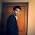Glad you found it useful. Cheers!

3.Santhosh Bandela15 August 2016 at 23:23

Excellent Work :
Attempted something through record macros but this one is excellent.
Thank you very much.

1.Thank you for stopping by my blog!

4.I LOVE IT!!
Thank you so much

5.THANK U SO MUCH

6.I keep getting an error on Sheets.Add(After:=Sheets(Sheets.Count)).Name = x.Value. I'm filtering column B and have data through column K.
Sub filter()
Application.ScreenUpdating = False
Dim x As Range
Dim rng As Range
Dim last As Long
Dim sht As String

'specify sheet name in which the data is stored
sht = "report"

'change filter column in the following code
last = Sheets(sht).Cells(Rows.Count, "B").End(xlUp).Row
Set rng = Sheets(sht).Range("A1:K" & last)

Sheets(sht).Range("B1:B" & last).AdvancedFilter Action:=xlFilterCopy, CopyToRange:=Range("AA1"), Unique:=True

For Each x In Range([AA2], Cells(Rows.Count, "AA").End(xlUp))
With rng
.AutoFilter
.AutoFilter Field:=2, Criteria1:=x.Value
.SpecialCells(xlCellTypeVisible).Copy

ActiveSheet.Paste
End With
Next x

' Turn off filter
Sheets(sht).AutoFilterMode = False

With Application
.CutCopyMode = False
.ScreenUpdating = True
End With

End Sub

7.I got it to work!!! Thank you!!! The issue was with one of the values I filtered; the characters exceeded the max of 30.

1.Glad to know it's working for you. Thanks!

2.Hi DB - It's not working for me. I know I'm close to your code but would appreciate your help. I'm filtering on Col. F and my data is till col. V.

Sub filter()
Application.ScreenUpdating = False
Dim x As Range
Dim rng As Range
Dim last As Long
Dim sht As String

'specify sheet name in which the data is stored
sht = "200012-MR09"

'change filter column in the following code
last = Sheets(sht).Cells(Rows.Count, "F").End(xlUp).Row
Set rng = Sheets(sht).Range("A1:V" & last)

Sheets(sht).Range("F1:F" & last).AdvancedFilter Action:=xlFilterCopy, CopyToRange:=Range("AA1"), Unique:=True

For Each x In Range([AA2], Cells(Rows.Count, "AA").End(xlUp))
With rng
.AutoFilter
.AutoFilter Field:=6, Criteria1:=x.Value
.SpecialCells(xlCellTypeVisible).Copy

ActiveSheet.Paste
End With
Next x

' Turn off filter
Sheets(sht).AutoFilterMode = False

With Application
.CutCopyMode = False
.ScreenUpdating = True
End With
End Sub

3.Thanks for the code bro...

8.I am getting the following error.
runtime error 9 subscript out of range
I am getting the error at line
"last = Sheets(sht).Cells(10, "D").End(xlUp).Row"

9.Can any one help me to resolve it. Thank you for your help in advance.

1.Have you specified your sheet name in this part of the code : sht = "DATA Sheet"? If yes, please save your file in the cloud drive and share the link with me to debug the code.

10.Hi, how can I use this for multiple sheets in a workbook to save all the filtered data in one.
Thanks.

11.It is not working. reason i need to filter data from certain col. and then paste the whole sheet in another worksheet. In above code you have pasted in col. AA and that too data for col. F onlt.thanks

1.It works. Did you follow the instruction properly? What error you are getting?

12.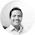Helo mr DB...
your code is work very well on the first time. But, when i ran twice or more, it gets error, I thinks its because the sheet with the same name already added. How to solve this..?

1.Add Sheets(x.Text).Delete after this line of code 'For Each x In Range([AA2], Cells(Rows.Count, "AA").End(xlUp))'. Hope it helps.

13.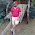hi deepanshu,
from the above data i would like filter each and every column with some condition then how it will be, in the above you filtered only f column right but i wanted to filter in each and every column with some specific condition, could pls tell me the code

14.Thanks it works for me

15.Hi Deepanshu...

Its great job you have done above... I found it very useful. Thank you very much for that.. I loved it... I wanted to make some small modification as per my requirements. Instead of creating new sheets in the same workbook, i want to create new workbook and copy the same data.. and name it in the same way as you did for worksheets... How can I do that..??

TYIA
Amaresh.A.R

1.I have the same need. Did you happen to find a solution for it?

16.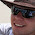Hi, thanks this is great. Would you perhaps know what to change the activesheet.paste to to be able to paste the formulas instead of the values?

1.ActiveSheet.Range("a1").PasteSpecial Paste:=xlFormulas

17.Can it be added SUM/Total of age column in each worksheet
For example in worksheet 1
name age adress contact org rank
richa 2 iii xyz prst 1
rekha 1 kkk xyz prst 1
seeta 1 kkk xyz prst 1
rahul 6 hhh xyz prst 1
Total 10

18.Can any one help me to resolve it. Thank you for your help in advance

19.1.I have added solution in the post. Hope it helps!

20.Deepanshu,
Thanks a lot for response,
Can you please specific the details, as i am unable to search-out the solution (what you have added related to my request i.e. SUM of age column in each sheet for example Total 10, in this post).
- Ravi

1.See 6th point under 'How to customize the above program'.

21.Yes,
It's done, thanks a lot SIR
- Ravi

22.Thank you so much

23.How do we customize the above code for the filter being one of the columns, say column C ?

I used the below code, but get an error.

'change filter column in the following code
last = Sheets(sht).Cells(Rows.Count, "C").End(xlUp).Row
Set rng = Sheets(sht).Range("A1:F" & last)

Sheets(sht).Range("C1:C" & last).AdvancedFilter Action:=xlFilterCopy, CopyToRange:=Range("AA1"), Unique:=True'change filter column in the following code
last = Sheets(sht).Cells(Rows.Count, "C").End(xlUp).Row
Set rng = Sheets(sht).Range("A1:F" & last)

Sheets(sht).Range("C1:C" & last).AdvancedFilter Action:=xlFilterCopy, CopyToRange:=Range("AA1"), Unique:=True

When I tried, I get the error "The exact range has a missing or illegal field name"

1.I'm having the same issue...

24.This comment has been removed by the author.

25.How can this be altered to have Pre-made sheets instead of generated.

26.hello
can I customise it to create a new workbook instead?

27.Hello, I have added the Sheets(x.Text).Delete after
For Each x In Range([AA2], Cells(Rows.Count, "AA").End(xlUp))
but I am getting a subscript out of range error on
Sheets(x.Text).Delete

1.2.Hi,I have uploaded the screenshots here.
( https://www.dropbox.com/sh/iar3yh2vl8ro0tl/AAA9gmdgyj2BLxAnb8bQGCvya?dl=0 )

3.By the way, I am using Excel 2016.

4.I have modified the code. Follow the 5th point under "How to Customize the above program". Cheers!

5.Thank you very much, Deepanshu! :)

28.Hi - thanks so much for the code. Is there any way of modifying so that only specific columns are copied and pasted? I have data in columns A-U but only want to copy U, E & F (table format with headers)

Have been going round in circles so any help much appreciated!

29.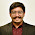I want to delete sheets when the data is removed, how can I do that?

30.Hi Bro,

Your code works Good. I would like to change simply for my requirements. What I try to do is, after the filtering of data, instead of copying the full active data which filtered, I want to copy the D column with only active cells except header and paste it in another sheet parallel with transpose of data

31.Hello,
What if I would like to filter the data based on criterias present in 2 different columns ?

32.Hi Deepa, thanks for sharing this. It is very helpful. One thing, what about if I wanted to have the same cells width and heights as the source. Thanks in advance.

33.CAN I FILTER THE SAME DATA WITH A COLUMN AS YOU SAID WITH F COLUMN

34.ANYWAY YOU DONE A GREAT JOB...

35.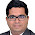Great , Simply Awesome. Saved a lot of manual work for me.

36.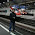Hi Db...code is excellent...it works with no header cell and only raw data. What if I have cell headers in the first two rows and want them copied as well in the new worksheets ? Second question is - I want filtered data with respect to two criteria but the name of the new worksheet is based on only one criteria ?

37.I came across this code while doing some research for filtering using VBA and it worked perfectly. I would like to know, how would I modify the VBA if I only require a few columns to be copied over into the new worksheet.

Example: The sheet I am filtering has 10 rows, but I only need to copy data from columns "A,C,D,E,F,H,I,J"

1.I have added it to this tutorial. Refer point #6. Thanks!

2.Much appreciated, thanks.

38.1.Me alegra que te haya resultado útil. Aclamaciones

39.Thanks!! absolutely gorgeous. I just needed something and approach like this. All variants are also interesting and very well explained. simple THANKS!!
Gracias!

1.40.My script below works well but I need to tweek it a bit.
Can you assist me with modifying the below vba.

1. I do not want to set any cell ranges only column range, so where I have "N1344", how do I change that to just "N" and make the script work?

2. I need to only copy columns A,C,D,E,F,H,I,J,K,L,M,N from my "Names" worksheet and paste into my "Backlog" worksheet. How do I modify the script to make it run? I tried similar to your #6 above and it didn't work.

Sub copyFilteredBacklog()
Dim rng As Range
Dim autofiltrng As Range
With Sheets("Names")
.Range("A1:N1344").AutoFilter field:=11, Criteria1:=">" & Range("N2").Value
End With
With Sheets("Names").AutoFilter.Range
On Error Resume Next
Set autofiltrng = .Offset(1, 0).Resize(.Rows.Count - 1, 1).SpecialCells(xlCellTypeVisible)
On Error GoTo 0

End With
If autofiltrng Is Nothing Then
MsgBox "no data available for copying!"
Else
Worksheets("Backlog").Range("A7:L1344").ClearContents
Set rng = Sheets("Names").AutoFilter.Range
rng.Offset(1, 0).Resize(rng.Rows.Count - 1).Copy Destination:=Sheets("Backlog").Range("A7")
Range("A7", Range("A7").End(xlDown).End(xlToRight)).Interior.Color = xlNone
Range("A7", Range("A7").End(xlDown).End(xlToRight)).Font.Color = vbBlack
End If
Sheets("Names").ShowAllData
End Sub

41.1. Add the following code after "Dim autofiltrng As Range" line
last = Sheets("Names").Cells(Rows.Count, "N").End(xlUp).Row

2. Replace Range("A1:N1344") with Range("A1:N" & last)

Can you show me the script for second point where you made changes as suggested above?

1.The below is the script, that copies the data to the "Backlog" sheet. But it copies all columns. I only want to copy columns A,C,D,E,F,H,I,J,K,L,M,N.

If autofiltrng Is Nothing Then
MsgBox "no data available for copying!"
Else
Worksheets("Backlog").Range("A7:L1344").ClearContents
Set rng = Sheets("Names").AutoFilter.Range
rng.Offset(1, 0).Resize(rng.Rows.Count - 1).Copy Destination:=Sheets("Backlog").Range("A7")
Range("A7", Range("A7").End(xlDown).End(xlToRight)).Interior.Color = xlNone
Range("A7", Range("A7").End(xlDown).End(xlToRight)).Font.Color = vbBlack
End If
Sheets("Names").ShowAllData
End Sub

42.Hi Deepanshu,

I am using your code to filter data and paste into a new Workbook. Your code is really helpful. But I am stuck at the below line

Am getting the error - method 'add' of object sheets failed. Below is my modified code. Could you please help me at the earliest?

Option Explicit

Sub filter()
Application.ScreenUpdating = False
Dim x As Range
Dim rng As Range
Dim rng1 As Range
Dim last As Long
Dim sht As String
Dim newBook As Excel.Workbook
Dim Workbk As Excel.Workbook

'Specify sheet name in which the data is stored
sht = "NEWDATA"

'Workbook where VBA code resides
Set Workbk = ThisWorkbook

'New Workbook
Workbk.Activate

'change filter column in the following code
last = Workbk.Sheets(sht).Cells(Rows.Count, "A").End(xlUp).Row

With Workbk.Sheets(sht)
Set rng = .Range("A1:E" & last)
End With

Workbk.Sheets(sht).Range("A1:A" & last).AdvancedFilter Action:=xlFilterCopy, CopyToRange:=Range("AA1"), Unique:=True
For Each x In Workbk.Sheets(sht).Range([AA2], Cells(Rows.Count, "AA").End(xlUp))

With rng
.AutoFilter
.AutoFilter Field:=1, Criteria1:=x.Value
.SpecialCells(xlCellTypeVisible).Copy

newBook.Activate
ActiveSheet.Paste
End With
Next x

' Turn off filter
Workbk.Sheets(sht).AutoFilterMode = False

With Application
.CutCopyMode = False
.ScreenUpdating = True
End With

End Sub

43.Helo, Is it possible to filter and copy data from one sheet and paste it in another but the column heads are different in second workbook. I need to copy from first sheet then paste in to corresponding columns in second work book

44.Hi the code is working good for me, but I have one requirement instead of copying 4unique sheets I want it for one unique value it should copy in the new sheet, can you please help me and also filter should be defined as one column contains values i.e in positive and in negetive integers and another column has open and closed staus here I want only open status that is with negetive interger.

45.Hi! Such a helpful thread, probably the most useful I've ever seen. Thank you for posting

I have tweaked the original VBA code you have provided to suit my spreadsheet (range A to O, filtering on A, Autofilter Field 1 ) but would like to have some code in which the user can input a value from Column A into a box, which then runs the original code to filter for that input, copy and pasta the data into a new workbook.
I have tried to use other codes from other sits but can't seem to figure this one out!

Any help would be greatly appreciated!

46.I am using your code to filter data and paste into a new worksheet. Your code is really helpful. But I am stuck at the below line. I have a filter on F and last is F itself

"last = Sheets(sht).Cells(Rows.Count, "F").End(xlUp).Row"

Sub filter()
Application.ScreenUpdating = False
Dim x As Range
Dim rng As Range
Dim last As Long
Dim sht As String

'specify sheet name in which the data is stored
sht = "Datasheet"

'change filter column in the following code
last = Sheets(sht).Cells(Rows.Count, "F").End(xlUp).Row
Set rng = Sheets(sht).Range("A1:F" & last)

Sheets(sht).Range("F1:F" & last).AdvancedFilter Action:=xlFilterCopy, CopyToRange:=Range("AA1"), Unique:=True

For Each x In Range([AA2], Cells(Rows.Count, "AA").End(xlUp))
With rng
.AutoFilter
.AutoFilter Field:=6, Criteria1:=x.Value
.SpecialCells(xlCellTypeVisible).Copy

ActiveSheet.Paste
End With
Next x

' Turn off filter
Sheets(sht).AutoFilterMode = False

With Application
.CutCopyMode = False
.ScreenUpdating = True
End With

End Sub

Next → ← Prev
Love this Post? Spread the Word!
Share